# Prism X

The prism with the edges of the lengths x cm, 2x cm and 3x cm has volume 20250 cm3. What is the area of surface of the prism?

Result

S =  4950 cm2

#### Solution:Leave us a comment of example and its solution (i.e. if it is still somewhat unclear...):Savage Math Hacker 21
i think that all math is great but cubes graphic is better.????????Dr Math
graphics fixed## Next similar examples:

1. Cuboid edges in ratioCuboid edges lengths are in ratio 2:4:6. Calculate their lengths if you know that the cuboid volume is 24576 cm3.
2. BallsThree metal balls with volumes V1=71 cm3 V2=78 cm3 and V3=64 cm3 melted into one ball. Determine it's surface area.
3. Sphere A2VSurface of the sphere is 241 mm2. What is its volume?
4. Cylinder - A&VThe cylinder has a volume 1287. The base has a radius 10. What is the area of surface of the cylinder?
5. Sphere fallHow many percent fall volume of sphere if diameter fall 10×?
6. Sphere growthHow many times grow volume of sphere if diameter rises 10×?
7. Theorem proveWe want to prove the sentence: If the natural number n is divisible by six, then n is divisible by three. From what assumption we started?
8. Square roots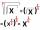What is equal to the product of the square roots of 295936?
9. One halfOne half of ? is: ?
10. CalculationHow much is sum of square root of six and the square root of 225?
11. PowersExpress the expression ? as the n-th power of the base 10.
12. PowerNumber ?. Find the value of x.
13. Holidays - on poolChildren's tickets to the swimming pool stands x € for an adult is € 2 more expensive. There was m children in the swimming pool and adults three times less. How many euros make treasurer for pool entry?
14. Root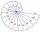Use law of square roots roots: ?
15. Unknown number 17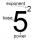Milada said, I am thinking of a number such that I evaluate expression x1/3, the value of the expression would be 5. Which number Milada thinking?
16. Unknown number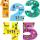Determine the unknown number, which double of its fourth square is equal the fifth its square.
17. Quotient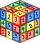Determine the quotient and the second member of the geometric progression where a3=10, a1+a2=-1,6 a1-a2=2,4.